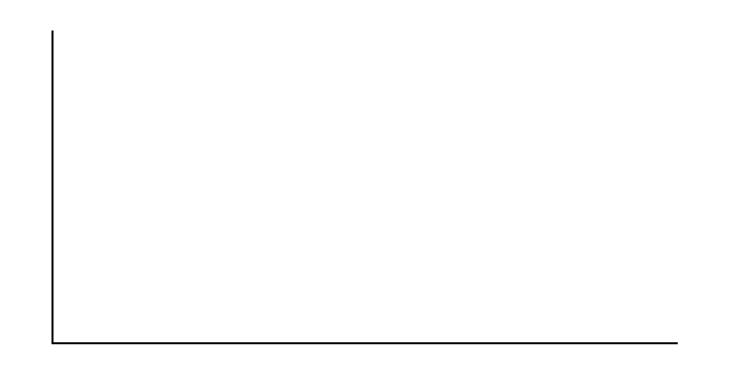The Chi-Square Distribution

# Lab 2: Chi-Square Test of Independence

Lab 2: Chi-Square Test of Independence

Class Time:

Names:

Student Learning Outcome
• The student will evaluate if there is a significant relationship between favorite type of snack and gender.
Collect the Data
1. Using your class as a sample, complete the following chart. Ask each other what your favorite snack is, then total the results.
Note

You may need to combine two food categories so that each cell has an expected value of at least five.

Favorite type of snack
sweets (candy & baked goods) ice cream chips & pretzels fruits & vegetables Total
male
female
Total
2. Looking at (Figure), does it appear to you that there is a dependence between gender and favorite type of snack food? Why or why not?

Hypothesis Test Conduct a hypothesis test to determine if the factors are independent:

1. H0: ________
2. Ha: ________
3. What distribution should you use for a hypothesis test?
4. Why did you choose this distribution?
5. Calculate the test statistic.
6. Find the p-value.
7. Sketch a graph of the situation. Label and scale the x-axis. Shade the area corresponding to the p-value.